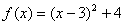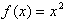Transformation

Function notation for the parent function of a quadratic is f(x) = x2. A function transformation takes the basic function f(x) and then "transforms" it, so that the graph will become more wide or narrow, reflect across the x-axis, or translate up, down, left or right.

To translate the graph of a quadratic function, you can use the vertex form, y = a(x - h) 2 + k.

• The h-value will translate the graph to the right when it is negative and to the left when it is positive.
• The k-value will translate the graph up when it is positive and down when it is negative.

Example The equation,, represents what translation of the parent function,?

Since the h -value is negative, the translation will be 3 units to the right. Since the k -value is positive, the translation will be up 4 units.

Listen